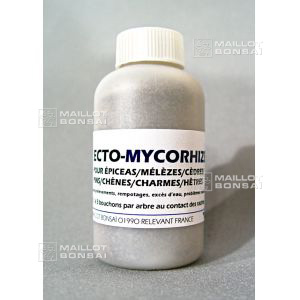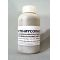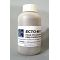##### The Japanese Bonsai specialist
Direct order Contact Help / Services Newsletter# Ecto mycorhize bonsai root treatment 100 gr

› Bonsai soil and fertiliser › Bonsai maintenanceref. : 5261

10,00

Available quantity : 71Order

###### Description

Small bottle 100Gr. This ECTO mycorhize treatment is meant for fertilising willow, hazelnut catkin trees, walnut, alder, birch, beech, fir, pine, cedar, larch, spruce, chestnut, myrtle, poplar, eucalyptus and oak.

It's especially good for bonsai with root problems such as excess water or root rot. It's highly recommended that you use this product when potting trees found in the forest (yamadori) that you want to train into a bonsai.

Benefits: mycorhize may reduce infection by bacteria and fungi that cause plant diseases. It can also help fix vital nutrients, promoting plant growth.

How to use: Use 1 to 3 capfuls per tree, make four or five holes in the earth, near the trunk and surface roots (not in the bottom of the pot), and add some powder to each. Re-cover the holes with earth afterwards.

Species:

Willow

Hazelnut catkin trees

Walnut

Alder

Birch

Beech

Fir

Pine

Cedar

Larch

Spruce

Chestnut

Myrtle

Poplar

Eucalyptus

Oak

#bonsai 6.6 #mycorhize 4.9 #root 4.4 #treatment 3.9 #trees 3.5 #ecto 3.4 #that 3.4 #maintenance 3.1 #eucalyptus 3 #chestnut 2.8

Formule
(( ROUND((CHAR_LENGTH(b.article_nom)-CHAR_LENGTH(REPLACE(b.article_nom, 'mycorhize', '')))/LENGTH('mycorhize')) + ROUND((CHAR_LENGTH(b.article_description)-CHAR_LENGTH(REPLACE(b.article_description, 'mycorhize', '')))/LENGTH('mycorhize')) ) * 4.9) + (( ROUND((CHAR_LENGTH(b.article_nom)-CHAR_LENGTH(REPLACE(b.article_nom, 'bonsai', '')))/LENGTH('bonsai')) + ROUND((CHAR_LENGTH(b.article_description)-CHAR_LENGTH(REPLACE(b.article_description, 'bonsai', '')))/LENGTH('bonsai')) ) * 4.6) + (( ROUND((CHAR_LENGTH(b.article_nom)-CHAR_LENGTH(REPLACE(b.article_nom, 'root', '')))/LENGTH('root')) + ROUND((CHAR_LENGTH(b.article_description)-CHAR_LENGTH(REPLACE(b.article_description, 'root', '')))/LENGTH('root')) ) * 4.4) + (( ROUND((CHAR_LENGTH(b.article_nom)-CHAR_LENGTH(REPLACE(b.article_nom, 'treatment', '')))/LENGTH('treatment')) + ROUND((CHAR_LENGTH(b.article_description)-CHAR_LENGTH(REPLACE(b.article_description, 'treatment', '')))/LENGTH('treatment')) ) * 3.9) + (( ROUND((CHAR_LENGTH(b.article_nom)-CHAR_LENGTH(REPLACE(b.article_nom, 'trees', '')))/LENGTH('trees')) + ROUND((CHAR_LENGTH(b.article_description)-CHAR_LENGTH(REPLACE(b.article_description, 'trees', '')))/LENGTH('trees')) ) * 3.5) + (( ROUND((CHAR_LENGTH(b.article_nom)-CHAR_LENGTH(REPLACE(b.article_nom, 'ecto', '')))/LENGTH('ecto')) + ROUND((CHAR_LENGTH(b.article_description)-CHAR_LENGTH(REPLACE(b.article_description, 'ecto', '')))/LENGTH('ecto')) ) * 3.4) + (( ROUND((CHAR_LENGTH(b.article_nom)-CHAR_LENGTH(REPLACE(b.article_nom, 'that', '')))/LENGTH('that')) + ROUND((CHAR_LENGTH(b.article_description)-CHAR_LENGTH(REPLACE(b.article_description, 'that', '')))/LENGTH('that')) ) * 3.4) + (( ROUND((CHAR_LENGTH(b.article_nom)-CHAR_LENGTH(REPLACE(b.article_nom, 'eucalyptus', '')))/LENGTH('eucalyptus')) + ROUND((CHAR_LENGTH(b.article_description)-CHAR_LENGTH(REPLACE(b.article_description, 'eucalyptus', '')))/LENGTH('eucalyptus')) ) * 3) + (( ROUND((CHAR_LENGTH(b.article_nom)-CHAR_LENGTH(REPLACE(b.article_nom, 'hazelnut', '')))/LENGTH('hazelnut')) + ROUND((CHAR_LENGTH(b.article_description)-CHAR_LENGTH(REPLACE(b.article_description, 'hazelnut', '')))/LENGTH('hazelnut')) ) * 2.8) + (( ROUND((CHAR_LENGTH(b.article_nom)-CHAR_LENGTH(REPLACE(b.article_nom, 'chestnut', '')))/LENGTH('chestnut')) + ROUND((CHAR_LENGTH(b.article_description)-CHAR_LENGTH(REPLACE(b.article_description, 'chestnut', '')))/LENGTH('chestnut')) ) * 2.8)

## Secure payment## Delivery

Our logistic partners :04 74 55 23 48
Pépinière MAILLOT-BONSAÏ
Le Bois Frazy
01990 RELEVANT - FRANCE
on appointment